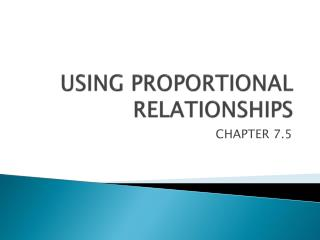DownloadDownload PresentationUSING PROPORTIONAL RELATIONSHIPS

USING PROPORTIONAL RELATIONSHIPS

Télécharger la présentationUSING PROPORTIONAL RELATIONSHIPS

- - - - - - - - - - - - - - - - - - - - - - - - - - - E N D - - - - - - - - - - - - - - - - - - - - - - - - - - -
Presentation Transcript

1. USING PROPORTIONAL RELATIONSHIPS CHAPTER 7.5

2. Helpful Hint Whenever dimensions are given in both feet and inches, you must convert them to either feet or inches before doing any calculations. INDIRECT MEASUREMENT • Indirect measurementis any method that uses formulas, similar figures, and/or proportions to measure an object. The following example shows one indirect measurement technique.

3. EXAMPLE 1 • Tyler wants to find the height of a telephone pole. He measured the pole’s shadow and his own shadow and then made a diagram. What is the height h of the pole?

4. EXAMPLE 1 CON’T Step 1 Convert the measurements to inches. AB = 7 ft 8 in. = (7  12) in. + 8 in. = 92 in. BC = 5 ft 9 in. = (5  12) in. + 9 in. = 69 in. FG = 38 ft 4 in. = (38  12) in. + 4 in. = 460 in. Step 2Find h. 92h = 69  460 h = 345 The height h of the pole is 345 inches, or 28 feet 9 inches.

5. EXAMPLE 2 • A student who is 5 ft 6 in. tall measured shadows to find the height LM of a flagpole. What is LM?

6. EXAMPLE 2 CON’T Step 1 Convert the measurements to inches. GH = 5 ft 6 in. = (5  12) in. + 6 in. = 66 in. JH = 5 ft = (5  12) in. = 60 in. NM = 14 ft 2 in. = (14  12) in. + 2 in. = 170 in. Step 2 Find h. 60(h) = 66  170 h = 187 The height of the flagpole is 187 in., or 15 ft. 7 in.

7. Remember! A proportion may compare measurements that have different units. SCALE DRAWING • A scale drawingrepresents an object as smaller than or larger than its actual size. The drawing’s scaleis the ratio of any length in the drawing to the corresponding actual length. For example, on a map with a scale of 1 cm : 1500 m, one centimeter on the map represents 1500 m in actual distance.

8. 9.6 cm 5.5 cm EXAMPLE 3 • Lady Liberty holds a tablet in her left hand. The tablet is 7.19 m long and 4.14 m wide. If you made a scale drawing using the scale 1 cm:0.75 m, what would be the dimensions to the nearest tenth? Set up proportions to find the length l and width w of the scale drawing. w  5.5 cm

9. SIMILARITY, PERIMETER, AREA

10. PROPORTIONAL PERIMETER AND AREA USING SIMILARITY RATIO

11. The similarity ratio of ∆LMN to ∆QRS is By the Proportional Perimeters and Areas Theorem, the ratio of the triangles’ perimeters is also , and the ratio of the triangles’ areas is EXAMPLE 4 • Given that ∆LMN ~∆QRT, find the perimeter P and area Aof ∆QRS.

12. EXAMPLE 4 CON’T • Perimeter Area 132A = (9.1)2(60) 13P = 36(9.1) A = 29.4 cm2 P = 25.2 The perimeter of ∆QRS is 25.2 cm, and the area is 29.4 cm2.

13. EXAMPLE 5 • ∆ABC ~ ∆DEF, BC = 4 mm, and EF = 12 mm. If P = 42 mm and A = 96 mm2 for ∆DEF, find the perimeter and area of ∆ABC. Perimeter Area 12P = 42(4) 122A = (4)2(96) P = 14 mm The perimeter of ∆ABC is 14 mm, and the area is 10.7 mm2.

14. HOMEWORK • WORKSHEET • P491 #’S 10-11 • P492 #’S 18-19